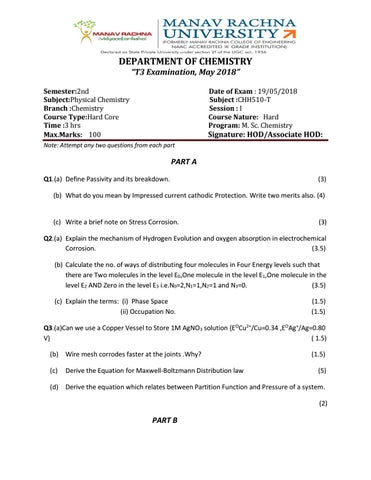DEPARTMENT OF CHEMISTRY “T3 Examination, May 2018” Semester:2nd Subject:Physical Chemistry Branch :Chemistry Course Type:Hard Core Time :3 hrs Max.Marks: 100

Date of Exam : 19/05/2018 Subject :CHH510-T Session : I Course Nature: Hard Program: M. Sc. Chemistry

Signature: HOD/Associate HOD:

Note: Attempt any two questions from each part

PART A Q1.(a) Define Passivity and its breakdown.

(3)

(b) What do you mean by Impressed current cathodic Protection. Write two merits also. (4)

(c) Write a brief note on Stress Corrosion.

(3)

Q2.(a) Explain the mechanism of Hydrogen Evolution and oxygen absorption in electrochemical Corrosion. (3.5) (b) Calculate the no. of ways of distributing four molecules in Four Energy levels such that there are Two molecules in the level E0,One molecule in the level E1,One molecule in the level E2 AND Zero in the level E3 i.e.N0=2,N1=1,N2=1 and N3=0. (3.5) (c) Explain the terms: (i) Phase Space (ii) Occupation No.

(1.5) (1.5)

Q3.(a)Can we use a Copper Vessel to Store 1M AgNO3 solution {EOCu2+/Cu=0.34 ,EOAg+/Ag=0.80 V} ( 1.5) (b) Wire mesh corrodes faster at the joints .Why? (c)

Derive the Equation for Maxwell-Boltzmann Distribution law

(1.5) (5)

(d) Derive the equation which relates between Partition Function and Pressure of a system. (2)

PART B

Q4.(a)What do you mean by Zwitter ionic /Amphoteric surfactant .Explain its properties with atleast two examples and applications. (5) (b)Explain the term Micellization.Discuss the thermodynamics of Micellization.

(5)

(c)What are the factors which effects CMC and Micellar Size .

(4)

(d)Explain Solubilization of Surfactant solution and also discuss the factors affecting Solubilization Capacity.

(6)

Q5(a)A polymer consist of 50% by weight of macromolecules of Molecular weight 15,000 &50% by weight of Macromolecules by mol.wt.65,000.Calculate the Number average( đ?&#x2018;&#x20AC;n) and weight average molecular weight.(đ?&#x2018;&#x20AC;đ?&#x2018;¤).

(3.5)

(b)How will you determine Molecular weight of polymers by Diffusion Methods and Light Scattering methods. (8) (c)Describe the Principle of working of Liquid Crystals cell.

(2.5)

(d)Explain in detail the types ,structure and applications of Liquid Crystal.

(6)

Q6.(a)Classify Polymers on the basis of Physical Properties with suitable examples.

(4)

(b)Define: (i)Degree of polymerization

(2)

(ii)Reverse micelle

(2)

(iii)Functionality

(2)

(c)How Thermodynamics of Micellar formation be explained on the basis of Mass Action model? (5) (d)How polymers be classified on the basis of Line Structure ,Polarity,mode of formation and Tacticity. (5)

PART C Q7.(a)Predict the mode of decay of (i)carbon-14 (ii)Xenon-118

(4)

(b)Discuss the stability of nucleus in terms of Neutron-proton ratio and Binding energy (6) (c)Differentiate between Controlled Nuclear fission and Uncontrolled nuclear fission

(4)

(d)Compare the nature of Îą,Î˛ and Ď&#x2019; radiations.

(6)

Q8.(a)In the neutron induced fission reaction of 92U235,one of the product is 37Rb95.During the fission three neutrons and another nuclide arealso produced.What is the other nuclide. (3.5) (b)Discuss the principle of Hydrogen Bomb.

(3.5)

(C)What is Binding Energy per nucleons ,explain it with graphical representation.

(3.5)

(d)Prove the relation 1amu = 931.5MeV

(3.5)

(e)What is the binding energy for 5B11 Nucleus of its mass defect0.08181 amu?

(2.5)

(f) Differentiate between fission products and fission Yield.

(3.5)

Q9.(a)Discuss the problems of carrying Nuclear Fusion in Nuclear Reactor

(3)

(b)83Bi214 decays to A by α –emission;A then Decays to B by β emission,which decays to C by another β emission.Element C decays to D by still another β emission,and D decays by α – emission to a stable isotope E.What is an E element? (4) (c)Explain the chief components of Nuclear Reactor,also discuss about” Light water nuclear power plant”. (6)

(d)Write sort notes on (i)Nuclear Isomerism

(2.5)

(ii)Stellar energy

(2)